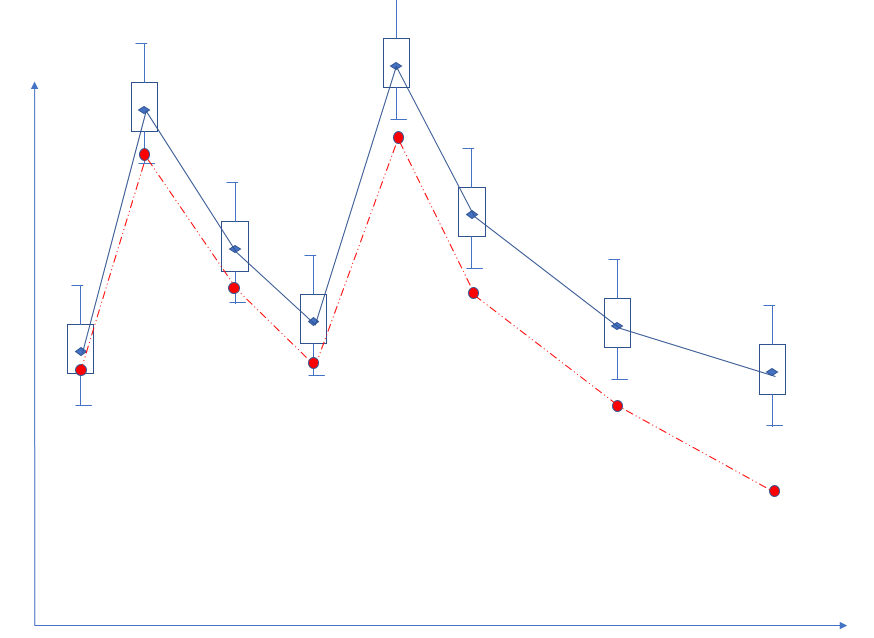## how to draw a figure includes box plot and scatter plotHi,

I want to draw this example figure in sas 9.3.

As you can see, there are two groups in the data, blue and red. blue one needs to draw the boxplot with mean connected, but the red one only needs to connect mean without box.

Can anyone help me with this?

Thanks.

1 ACCEPTED SOLUTION

Accepted SolutionsKsharp
Super User

## Re: how to draw a figure includes box plot and scatter plot

``````proc summary data=sashelp.class nway;
class sex;
var weight;
output out=temp mean=mean;
run;

data class;
set sashelp.class(keep=sex height) temp;
run;

proc sgplot data=class;
vbox height/ category=sex connect=mean;
series x=sex y=mean/markerattrs=(symbol=circlefilled) markers lineattrs=(pattern=dash color=red);
run;``````
7 REPLIES 7

## Re: how to draw a figure includes box plot and scatter plot

Here's an example of how you can do this:

https://communities.sas.com/t5/Graphics-Programming/Boxplot-multiple-variable-connecting-stats-using...

Since the code uses SASHELP.CARS you should be able to run the code directly and test it out.

``````proc sort data=sashelp.cars out=cars;
by origin;
run;

proc sgplot data=cars nocycleattrs noautolegend;
by origin;
vbox mpg_city / category=type connect=q1;
vbox mpg_city / category=type nofill connect=q3;
run;``````

## Re: how to draw a figure includes box plot and scatter plot

Thanks for your quick reply. But this is not what i want, this is an example for "Box Plot with Multiple Connect Lines".

What I need is the two groups are showing in different formats, blue group is a box plot, but the red group only needs to connect mean, does not need box. And i want these two groups in one figure.

Thanks.

## Re: how to draw a figure includes box plot and scatter plot

Ok, can you show your current data structure and anything you've tried so far. That code should have given you an idea of how to proceed-basically you can have multiple graphing statements in a plot.

SAS 9.3 does have more limitations though, which exact version are you on? 9.3 earlier versions are really limited for example while the ones closer to 9.4 are more up to date. By the way, 9.3 is about 7 years old or more, upgrades are usually included with SAS license so if you can upgrade I'd highly recommend it.

## Re: how to draw a figure includes box plot and scatter plot

Thanks,

I tried the following code:

proc sgplot data=subset noautolegend nocycleattrs;
vbox Result / category=time group=status connect=mean;
run;

My question is: if I add group comment after / , then the two groups are both shown in box plot. I do not know how to separate these two groups with different formats.

I wonder if there is an easy way to do it.

## Re: how to draw a figure includes box plot and scatter plot

I would put them in different variables and add a separate series statement for the second series. I think that's a bit harder in 9.3 but doable.Ksharp
Super User

## Re: how to draw a figure includes box plot and scatter plot

``````proc summary data=sashelp.class nway;
class sex;
var weight;
output out=temp mean=mean;
run;

data class;
set sashelp.class(keep=sex height) temp;
run;

proc sgplot data=class;
vbox height/ category=sex connect=mean;
series x=sex y=mean/markerattrs=(symbol=circlefilled) markers lineattrs=(pattern=dash color=red);
run;``````

## Re: how to draw a figure includes box plot and scatter plot

Thanks very much!

Discussion stats
• 7 replies
• 508 views
• 2 likes
• 3 in conversation